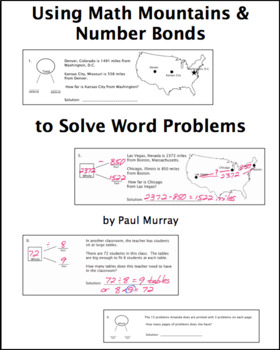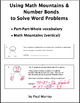Picturing the Part-Part-Whole Relationship with Math Mountains & Number BondsSubject
Resource Type
Common Core State Standards
Product Rating
File Type

Compressed Zip File

Be sure that you have an application to open this file type before downloading and/or purchasing.

45 MB|24 pages of student work
Share
Product Description
Understanding the relationships between quantities is important in making sense of problems. We all struggle with helping students as they read and attempt to comprehend problems, and we all know the frustration of seeing students give a solution that can’t possibly work because it is counter to the logic of the problem.

Understanding the relationship between the relative size of the numbers contained in a problem helps define the range of possible solutions. If I have \$20 and want to buy a book for \$9, I will not have \$29 left. If we cut a pizza into 12 slices and we want 2 slices for each person, we cannot feed 24 people. How to identify the parts and the wholes?

We have a very simple, comprehensible tool for visually expressing the relationship between parts and wholes in the Math Mountain, or Number Bonds. These are as useful for multiplication and division as they are for addition and subtraction.

It‘s clear to almost all students by the time they’re in third grade that the big number is by itself, whether on the top or one side, and the other 2 numbers combine in some way to make that big number. We can use that understanding to help students monitor what they do with the numbers they encounter in a problem. We focus on the known, rather than the unknown, numbers to help identify that big number. This in turn suggests which operation to use on the known numbers.

A simple sequence of questions governs how we plug the numbers in a problem into the Math Mountain, and how we can identify the operation. There are 24 pages of (mostly) problems. The problems are divided into three sections, one containing addition/subtraction problems, a second containing multiplication/division problems, and a third section of problems using all four operations.

Because there are several varieties of Math Mountain, number bonds, and terminologies in use, all these problems are presented in four different formats.

1. Math Mountains using the terms addend, factor, and total.

2. Math Mountains using the terms part and whole.

3. Number bonds using the terms addend, factor, and total.

4. Number bonds using the terms part and whole.

The number bonds have the total on the left and the parts on the right. Examples of each of the formats are shown in the thumbnails and the Preview File.

The magnitude of the numbers is geared toward 3rd and 4th grades. There are problems involving addition and subtraction of 2, 3, and 4-digit numbers. Multiplication and division are limited to basic facts with a few 2-digit quotients. The emphasis throughout is on identifying the correct operations, so the computational requirements are not intended to be an obstacle.

A Smart Notebook 14 file is included.

Common Core Standards:

2.OA.1: “Use addition and subtraction within 100 to solve one- and two-step
word problems….”

3.OA.3: “Use multiplication and division within 100 to solve word problems in
situations involving equal groups, arrays, and measurement quantities...”

4.OA.2: “Multiply or divide to solve word problems involving multiplicative
comparison…”

Subject: Math, Problem solving

Level: Grades 3 – 5

Length/Duration: 24 pages of student work, many problems, several days

Total Pages
24 pages of student work
Included
Teaching Duration
4 days
Report this Resource to TpT
Reported resources will be reviewed by our team. Report this resource to let us know if this resource violates TpT’s content guidelines.